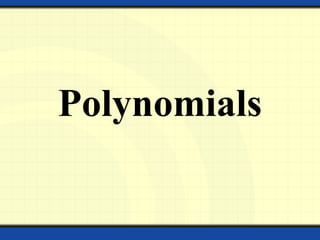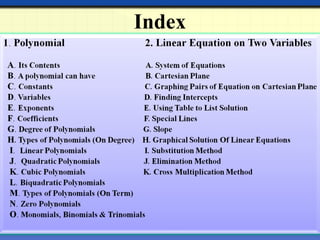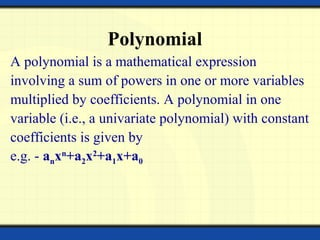Diese Präsentation wurde erfolgreich gemeldet.

# Polynomials And Linear Equation of Two Variables

Anzeige
Anzeige
Anzeige
Anzeige
Anzeige
Anzeige
Anzeige
Anzeige
Anzeige
Anzeige
Anzeige×

1 von 47 Anzeige

# Polynomials And Linear Equation of Two Variables

A complete description of polynomials and also various methods to solve the Linear equation of two variables by substitution, cross multiplication and elimination methods.
For polynomials it also contains the description of monomials, binomials etc.

A complete description of polynomials and also various methods to solve the Linear equation of two variables by substitution, cross multiplication and elimination methods.
For polynomials it also contains the description of monomials, binomials etc.

Anzeige
Anzeige

### Polynomials And Linear Equation of Two Variables

1. 1. Polynomials
2. 2. Index
3. 3. Polynomial A polynomial is a mathematical expression involving a sum of powers in one or more variables multiplied by coefficients. A polynomial in one variable (i.e., a univariate polynomial) with constant coefficients is given by e.g. - anxn +a2x2 +a1x+a0
4. 4. Polynomial Can Have A polynomial can have: Constants Variables Exponents Coefficients
5. 5. Constants In mathematics, a constant is a non- varying value, i.e. completely fixed or fixed in the context of use. The term usually occurs in opposition to variable (i.e. variable quantity), which is a symbol that stands for a value that may vary. For e.g. 2x2 +11y-22=0, here -22 is a constant.
6. 6. Variables In mathematics, a variable is a value that may change within the scope of a given problem or set of operations. In contrast, a constant is a value that remains unchanged, though often unknown or undetermined. For e.g. 10x2 +5y=2, here x and y are variable.
7. 7. Exponents Exponents are sometimes referred to as powers and means the number of times the 'base' is being multiplied. In the study of algebra, exponents are used frequently. For e.g.-
8. 8. Coefficients For other uses of this word, see coefficient (disambiguation).In mathematics, a coefficient is a multiplicative factor in some term of an expression (or of a series); it is usually a number, but in any case does not involve any variables of the expression. For e.g.- 7x2 − 3xy + 15 + y Here 7, -3, 1 are the coefficients of x2 , xy and y respectively.
9. 9. Degree of Polynomials The degree of a polynomial is the highest degree for a term. The degree of a term is the sum of the powers of each variable in the term. The word degree has for some decades been favoured in standard textbooks. In some older books, the word order is used. For e.g.- The polynomial 3 − 5x + 2x5 − 7x9 has degree 9.
10. 10. Types Of Polynomial Polynomials classified by degree –
11. 11. Linear Polynomials In a different usage to the above, a polynomial of degree 1 is said to be linear, because the graph of a function of that form is a line. For e.g.- 2x+1 11y +3
12. 12. Quadratic Polynomials In mathematics, a quadratic polynomial or quadratic is a polynomial of degree two, also called second-order polynomial. That means the exponents of the polynomial's variables are no larger than 2. For e.g.- x2 − 4x + 7 is a quadratic polynomial, while x3 − 4x + 7 is not.
13. 13. Cubic Polynomials Cubic polynomial is a polynomial of having degree of polynomial no more than 3 or highest degree in the polynomial should be 3 and should not be more or less than 3. For e.g.- x3 + 11x = 9x2 + 55 x3 + x2 +10x = 20
14. 14. Biquadratic Polynomials Biquadratic polynomial is a polynomial of having degree of polynomial is no more than 4 or highest degree in the polynomial is not more or less than 4. For e.g.- 4x4 + 5x3 – x2 + x - 1 9y4 + 56x3 – 6x2 + 9x + 2
15. 15. Types Of Polynomial Polynomial can be classified by number of non-zero term
16. 16. Zero Polynomials The constant polynomial whose coefficients are all equal to 0. The corresponding polynomial function is the constant function with value 0, also called the zero map. The degree of the zero polynomial is undefined, but many authors conventionally set it equal to -1 or ∞.
17. 17. Monomial, Binomial & Trinomial Monomial:- A polynomial with one term. E.g. - 5x3 , 8, and 4xy. Binomial:- A polynomial with two terms which are not like terms. E.g. - 2x – 3, 3x5 +8x4 , and 2ab – 6a2 b5 . Trinomial:- A polynomial with three terms which are not like terms. E.g. - x2 + 2x - 3, 3x5 - 8x4 + x3 , and a2 b + 13x + c.
18. 18. LINEAR EQUATION ON TWO VARIABLES
19. 19. A pair of linear equations in two variables is said to form a system of simultaneous linear equations. For Example, 2x – 3y + 4 = 0 x + 7y – 1 = 0 Form a system of two linear equations in variables x and y. System of Equations or Simultaneous Equations
20. 20. The general form of a linear equation in two variables x and y is ax + by + c = 0 , a ≠ 0, b ≠ 0, where a, b and c being real numbers. A solution of such an equation is a pair of values, one for x and the other for y, which makes two sides of the equation equal. Every linear equation in two variables has infinitely many solutions which can be represented on a certain line.
21. 21. Cartesian Plane x- axis y-axis Quadrant I (+,+) Quadrant II ( - ,+) Quadrant IV (+, - ) Quadrant III ( - , - ) origin
22. 22. Graphing Ordered Pairs on a Cartesian Plane x- axis y-axis 1) Begin at the origin. 2) Use the x-coordinate to move right (+) or left (-) on the x-axis. 3) From that position move either up(+) or down(-) according to the y- coordinate . 4) Place a dot to indicate a point on the plane. Examples: (0, -4) (6, 0) (-3, -6) (6,0) (0,-4) (-3, -6)
23. 23. Graphing More Ordered Pairs from our Table for the equation x y (3,-2) (3/2,-3) (-6, -8) 2x – 3y = 12 •Plotting more points we see a pattern. •Connecting the points a line is formed. •We indicate that the pattern continues by placing arrows on the line. •Every point on this line is a solution of its equation.
24. 24. Graphing Linear Equations in Two Variables  The graph of any linear equation in two variables is a straight line.  Finding intercepts can be helpful when graphing.  The x-intercept is the point where the line crosses the x- axis.  The y-intercept is the point where the line crosses the y- axis.  On our previous graph, y = 2x – 3y = 12, find the intercepts. y x
25. 25. Graphing Linear Equations in Two Variables • On our previous graph, y = 2x – 3y = 12, find the intercepts. • The x-intercept is (6,0). • The y-intercept is (0,-4). y x
26. 26. Finding INTERCEPTS To find the x-intercept: Plug in ZERO for y and solve for x. 2x – 3y = 12 2x – 3(0) = 12 2x = 12 x = 6 Thus, the x-intercept is (6,0). To find the y-intercept: Plug in ZERO for x and solve for y. 2(0) – 3y = 12 2(0) – 3y = 12 -3y = 12 y = -4 Thus, the y-intercept is (0,-4).
27. 27. Using Tables to List Solutions  For an equation we can list some solutions in a table.  Or, we may list the solutions in ordered pairs . {(0,-4), (6,0), (3,-2), ( 3/2, -3), (-3,-6), (-6,-8), … } 2 3 12x y− =
28. 28. Special Lines y = # is a horizontal line x = # is a vertical line y x y x
29. 29. Given 2 collinear points, find the slope. Find the slope of the line containing (3,2) and (-1,5). ( ) 2 1 2 1 2 5 3 3 1 4 y y m x x − − − = = = − − − Slop e
30. 30. GRAPHICAL SOLUTIONS OF A LINEAR EQUATION Let us consider the following system of two simultaneous linear equations in two variable. 2x – y = -1 3x + 2y = 9 Here we assign any value to one of the two variables and then determine the value of the other variable from the given equation.
31. 31. For the equation 2x –y = -1 ---(1) 2x +1 = y Y = 2x + 1
32. 32. XX´ Y Y ´ (2,5) (-1,6) (0,3)(0,1)
33. 33. ALGEBRAIC METHODS OF SOLVING SIMULTANEOUS LINEAR EQUATIONS The most commonly used algebraic methods of solving simultaneous linear equations in two variables are 1. Method of elimination by substitution 2. Method of elimination by equating the coefficient 3. Method of Cross- multiplication
34. 34. ELIMINATION BY SUBSTITUTION STEPS: Obtain the two equations. Let the equations be a1x + b1y + c1 = 0 ----------- (I ) a2x + b2y + c2 = 0 ----------- (II) Choose either of the two equations, say (I ) and find the value of one variable , say ‘y’ in terms of x Substitute the value of y, obtained in the previous step in equation (II) to get an equation in x
35. 35. SUBSTITUTION METHOD Solve the equation obtained in the previous step to get the value of x. Substitute the value of x and get the value of y. Let us take an example x + 2y = -1 ------------------ (I) 2x – 3y = 12 -----------------(II)
36. 36. SUBSTITUTION METHOD x + 2y = -1 x = -2y -1 ------- (III) Substituting the value of x in equation (II), we get 2x – 3y = 12 2 ( -2y – 1) – 3y = 12 - 4y – 2 – 3y = 12 - 7y = 14 , y = -2 ,
37. 37. SUBSTITUTION METHOD Putting the value of y in eq. (III), we get x = - 2y -1 x = - 2 x (-2) – 1 x = 4 - 1 x = 3 Hence the solution of the equation is ( 3, - 2 )
38. 38. ELIMINATION METHOD In this method, we eliminate one of the two variables to obtain an equation in one variable which can easily be solved. Putting the value of this variable in any of the given equations, the value of the other variable can be obtained. For example: we want to solve, 3x + 2y = 11 2x + 3y = 4
39. 39. Let 3x + 2y = 11 --------- (I) 2x + 3y = 4 ---------(II) Multiply 3 in equation (I) and 2 in equation (ii) and subtracting eq. iv from iii, we get
40. 40. putting the value of y in equation (II) we get, 2x + 3y = 4 2 x 5 + 3y = 4 10 + 3y = 4 3y = 4 – 10 3y = - 6 y = - 2 Hence, x = 5 and y = -2
41. 41. Cross Multiplication Method In elementary arithmetic, given an equation between two fractions or rational expressions, one can cross-multiply to simplify the equation or determine the value of a variable. For an equation like the following:
42. 42. Cross Multiplication Method Now, Then, ad = bc Let us now take some examples, 2x+3y=46 & 3x+5y=74
43. 43. Cross Multiplication Method 2x+3y=46, i.e., 2x+3y-46=0 3x+5y=74, i.e., 3x+5y-74=0 We know that equation for this method is- So, a1=2, b1=3, c1=-46 & a2=3, b2=5, c2=-74
44. 44. Cross Multiplication Method
45. 45. Cross Multiplication Method Taking eq. 1st and eq. 3rd together, we get
46. 46. Cross Multiplication Method Taking eq. 2nd and eq. 3rd together, we get So, value of x and y by cross multiplication method is 8 and 10 respectively.